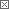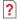Previous in Forum: What is the Stack Crack ? Next in Forum: Transformers in SeriesActive Contributor

Join Date: Mar 2010
Posts: 13

#Sound End Reflection Factor

08/19/2010 9:09 PM

Hello,

Did anyone know what is end reflection factor?

I had just visit a 3rd partie that using a reverberant sound room to measure the test unit sound power. They calculate the room absorption constant by using a know RSS(reference sound source). Then measure the test unit sound pressure and adding the room absorption constant plus the end reflection factor for each frequency, the sound power calculated. what do the end reflection factor here mean?

From what i know, the Lw = Lp + A

where Lw = sound power level

Lp = Sound pressure level

and A = Room absorption constant

Interested in this topic? By joining CR4 you can "subscribe" to